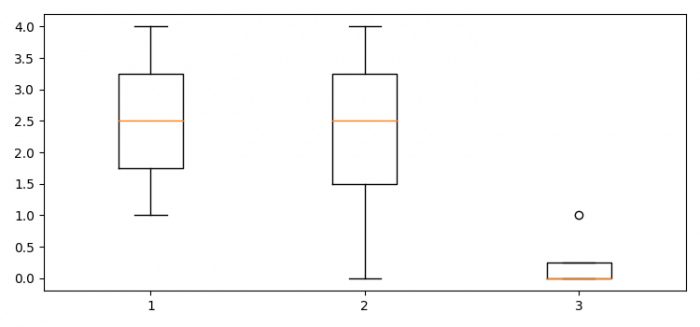# Boxplot with variable length data in Matplotlib

To make a boxplot with variable length data in Matplotlib, we can take the following steps −

• Set the figure size and adjust the padding between and around the subplots.
• Make a list of data points.
• Make a box and whisker plot using boxplot() method.
• To display the figure, use show() method.

## Example

from matplotlib import pyplot as plt

plt.rcParams["figure.figsize"] = [7.50, 3.50]
plt.rcParams["figure.autolayout"] = True

data = [[2, 4, 1, 3], [0, 4, 3, 2], [0, 0, 1, 0]]

plt.boxplot(data)

plt.show()

## Output